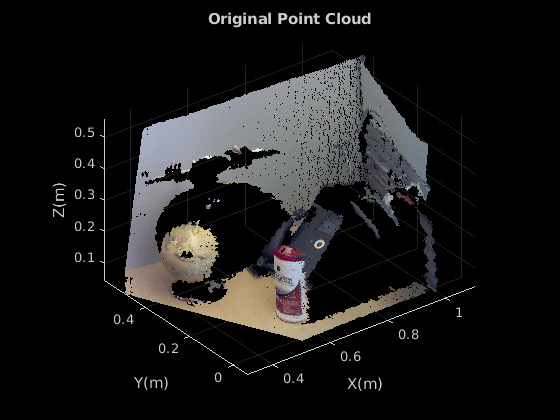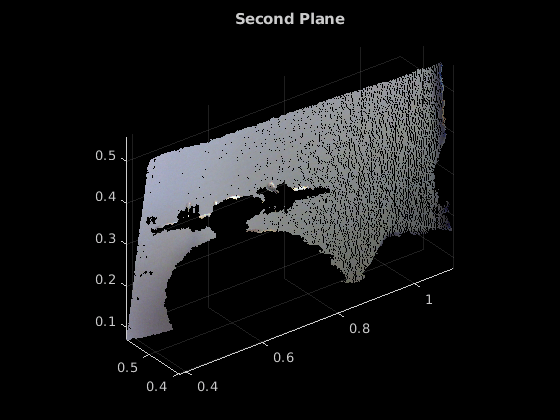pcfitplane

Fit plane to 3-D point cloud

Description

model = pcfitplane(ptCloudIn,maxDistance) fits a plane to a point cloud that has a maximum allowable distance from an inlier point to the plane. The function returns a geometrical model that describes the plane.

This function uses the M-estimator SAmple Consensus (MSAC) algorithm to find the plane. The MSAC algorithm is a variant of the RANdom SAmple Consensus (RANSAC) algorithm.

model = pcfitplane(ptCloudIn,maxDistance,referenceVector) fits a plane to a point cloud that has additional orientation constraints specified by the 1-by-3 referenceVector input.

example

model = pcfitplane(ptCloudIn,maxDistance,referenceVector,maxAngularDistance) fits a plane to a point cloud that has a specified maximum angular distance.

[model,inlierIndices,outlierIndices] = pcfitplane(ptCloudIn,maxDistance) additionally returns the linear indices to the inlier and outlier points in the point cloud input.

[___,meanError] = pcfitplane(ptCloudIn,maxDistance) additionally returns the mean error of the distance of inlier points to the model, using any of the preceding syntaxes.

example

[___] = pcfitplane(ptCloudIn,maxDistance,Name,Value) uses additional options specified by one or more Name,Value pair arguments.

Examples

collapse all

Display and label the point cloud.

figure
pcshow(ptCloud)
xlabel('X(m)')
ylabel('Y(m)')
zlabel('Z(m)')
title('Original Point Cloud')Set the maximum point-to-plane distance (2cm) for plane fitting.

maxDistance = 0.02;

Set the normal vector of the plane.

referenceVector = [0,0,1];

Set the maximum angular distance to 5 degrees.

maxAngularDistance = 5;

Detect the first plane, the table, and extract it from the point cloud.

[model1,inlierIndices,outlierIndices] = pcfitplane(ptCloud,...
maxDistance,referenceVector,maxAngularDistance);
plane1 = select(ptCloud,inlierIndices);
remainPtCloud = select(ptCloud,outlierIndices);

Set the region of interest to constrain the search for the second plane, left wall.

roi = [-inf,inf;0.4,inf;-inf,inf];
sampleIndices = findPointsInROI(remainPtCloud,roi);

Detect the left wall and extract it from the remaining point cloud.

[model2,inlierIndices,outlierIndices] = pcfitplane(remainPtCloud,...
maxDistance,'SampleIndices',sampleIndices);
plane2 = select(remainPtCloud,inlierIndices);
remainPtCloud = select(remainPtCloud,outlierIndices);

Plot the two planes and the remaining points.

figure
pcshow(plane1)
title('First Plane')figure
pcshow(plane2)
title('Second Plane')figure
pcshow(remainPtCloud)
title('Remaining Point Cloud')Input Arguments

collapse all

Point cloud, specified as a pointCloud object.

Maximum distance from an inlier point to the plane, specified as a scalar value. Specify the distance in units that are consistent with the units you are using for the point cloud.

Data Types: single | double

Reference orientation constraint, specified as a 1-by-3 vector.

Data Types: single | double

Maximum absolute angular distance between the normal vector of the fitted plane and the reference orientation, specified as a scalar value in degrees.

Data Types: single | double

Name-Value Arguments

Specify optional comma-separated pairs of Name,Value arguments. Name is the argument name and Value is the corresponding value. Name must appear inside quotes. You can specify several name and value pair arguments in any order as Name1,Value1,...,NameN,ValueN.

Example: 'SampleIndices',[].

Linear indices of points to sample in the input point cloud, specified as the comma-separated pair consisting of 'SampleIndices' and a column vector. An empty vector means that all points are candidates to sample in the RANSAC iteration to fit the plane. When you specify a subset, only points in the subset are sampled to fit a model.

Providing a subset of points can significantly speed up the process and reduce the number of trials. You can generate the indices vector using the findPointsInROI method of the pointCloud object.

Maximum number of random trials for finding inliers, specified as the comma-separated pair consisting of 'MaxNumTrials' and a positive integer. Increasing this value makes the output more robust but adds additional computations.

Confidence percentage for finding maximum number of inliers, specified as the comma-separated pair consisting of 'Confidence' and a numeric scalar, in the range [0 100]. Increasing this value makes the output more robust but adds additional computations.

Output Arguments

collapse all

Geometric model of plane, returned as a planeModel object.

When the input point cloud does not contain enough valid points, or when the function cannot find enough inlier points, the coefficients for the output model are set to zero.

Linear indices of inlier points within the input point cloud, returned as a column vector.

Linear indices of outlier points within the input point cloud, returned as a column vector.

Mean error of the distance of inlier points to the model, returned as a scalar value.

 Torr, P. H. S., and A. Zisserman. “MLESAC: A New Robust Estimator with Application to Estimating Image Geometry.” Computer Vision and Image Understanding. 2000.